# Octacode

(diff) ← Older revision | Latest revision (diff) | Newer revision → (diff)
Led by modulation considerations, G.D. Forney and M.D. Trott discovered in October 1992 that the Nordstrom–Robinson code was obtained by Gray mapping (cf. also Gray code) a certain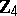code of lengthand minimum Lee distance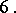Seeing the parity-check matrix of that code,NJ.A. Sloane identified this code with the octacode [a4], which had turned up already in one of the "holy constructions" of the Leech lattice [a2], Chapt. 24, in particular in the construction based on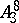. The Leech lattice, the conjecturally densest sphere packing in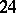dimensions, can be built up from a product of eight copies of the face-centred cubic lattice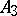, the conjecturally densest sphere packing in three dimensions. The quotient ofin its dual lattice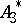is a cyclic group of order, and so to get the Leech lattice fromone needs a code of lengthover.
The preceding matrix shows that the octacode is an extended cyclic code with parity-check polynomial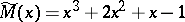, which reduced moduloyields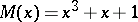, which is the generator matrix of the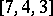binary Hamming code. It is indeed both the first quaternary Kerdock code and the first quaternary Preparata code [a5] (cf. also Kerdock and Preparata codes), and as such it is self-dual [a3]. It is indeed of type II, i.e. the Euclidean weight of its words is multiple of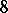; the attached lattice is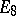, the unique even unimodular lattice in dimension[a1]. Its residue code modulois the doubly even binary self-dual code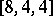.